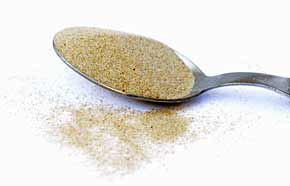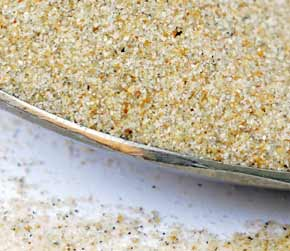﻿ Beach Sand 1 pound weight to cubic centimeters converter

beach sand conversion

Amount: 1 pound (lb) of weight Equals: 296.62 cubic centimeters (cc - cm3) in volume

Converting pound to cubic centimeters value in the beach sand units scale.

TOGGLE :   from cubic centimeters into pounds in the other way around.

beach sand from pound to cubic centimeter Conversion Results:

Enter a New pound Amount of beach sand to Convert From

* Whole numbers, decimals or fractions (ie: 6, 5.33, 17 3/8)
* Precision is how many numbers after decimal point (1 - 9)

Enter Amount :
Decimal Precision :

CONVERT :   between other beach sand measuring units - complete list.

Conversion calculator for webmasters.

Beach sand weight vs. volume units

Beach sand has quite high density, it's heavy and it easily leaks into even tiny gaps or other opened spaces. No wonder it absorbs and conducts heat energy from the sun so well. However, this sand does not have the heat conductivity as high as glass does, or fireclay and firebricks, or dense concrete. A fine beach sand in dry form was used for taking these measurements.Convert beach sand measuring units between pound (lb) and cubic centimeters (cc - cm3) but in the other reverse direction from cubic centimeters into pounds.

 conversion result for beach sand: From Symbol Equals Result To Symbol 1 pound lb = 296.62 cubic centimeters cc - cm3

Converter type: beach sand measurements

This online beach sand from lb into cc - cm3 converter is a handy tool not just for certified or experienced professionals.

First unit: pound (lb) is used for measuring weight.
Second: cubic centimeter (cc - cm3) is unit of volume.

beach sand per 296.62 cc - cm3 is equivalent to 1 what?

The cubic centimeters amount 296.62 cc - cm3 converts into 1 lb, one pound. It is the EQUAL beach sand weight value of 1 pound but in the cubic centimeters volume unit alternative.

How to convert 2 pounds (lb) of beach sand into cubic centimeters (cc - cm3)? Is there a calculation formula?

First divide the two units variables. Then multiply the result by 2 - for example:
296.62069709652 * 2 (or divide it by / 0.5)

QUESTION:
1 lb of beach sand = ? cc - cm3

1 lb = 296.62 cc - cm3 of beach sand

Other applications for beach sand units calculator ...

With the above mentioned two-units calculating service it provides, this beach sand converter proved to be useful also as an online tool for:
1. practicing pounds and cubic centimeters of beach sand ( lb vs. cc - cm3 ) measuring values exchange.
2. beach sand amounts conversion factors - between numerous unit pairs variations.
3. working with mass density - how heavy is a volume of beach sand - values and properties.

International unit symbols for these two beach sand measurements are:

Abbreviation or prefix ( abbr. short brevis ), unit symbol, for pound is:
lb
Abbreviation or prefix ( abbr. ) brevis - short unit symbol for cubic centimeter is:
cc - cm3

One pound of beach sand converted to cubic centimeter equals to 296.62 cc - cm3

How many cubic centimeters of beach sand are in 1 pound? The answer is: The change of 1 lb ( pound ) weight unit of beach sand measure equals = to volume 296.62 cc - cm3 ( cubic centimeter ) as the equivalent measure within the same beach sand substance type.

In principle with any measuring task, switched on professional people always ensure, and their success depends on, they get the most precise conversion results everywhere and every-time. Not only whenever possible, it's always so. Often having only a good idea ( or more ideas ) might not be perfect nor good enough solution. If there is an exact known measure in lb - pounds for beach sand amount, the rule is that the pound number gets converted into cc - cm3 - cubic centimeters or any other beach sand unit absolutely exactly.

Conversion for how many cubic centimeters ( cc - cm3 ) of beach sand are contained in a pound ( 1 lb ). Or, how much in cubic centimeters of beach sand is in 1 pound? To link to this beach sand pound to cubic centimeters online converter simply cut and paste the following.
The link to this tool will appear as: beach sand from pound (lb) to cubic centimeters (cc - cm3) conversion.

I've done my best to build this site for you- Please send feedback to let me know how you enjoyed visiting.